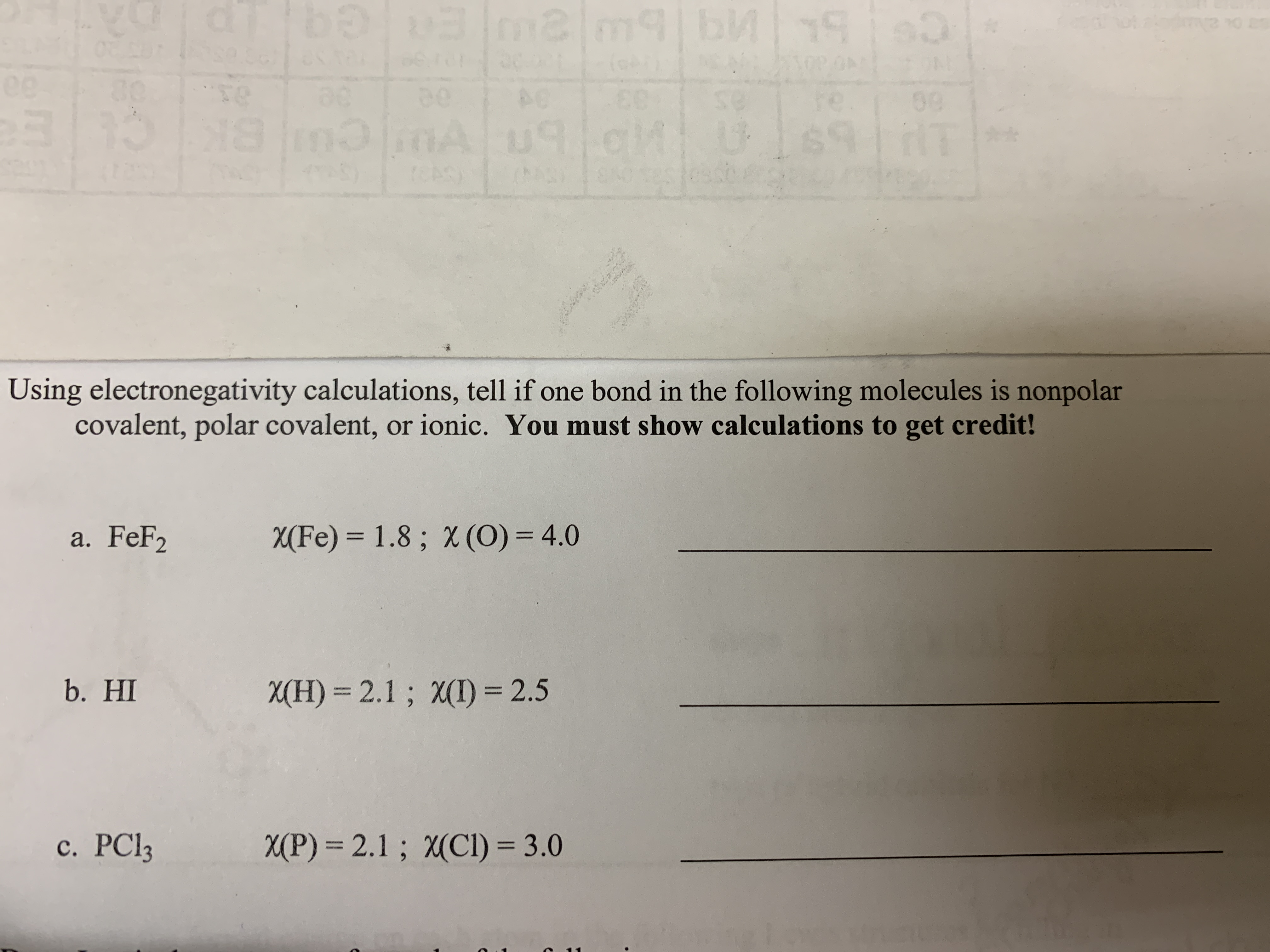# baamlm9|bM30te00BK17Using electronegativity calculations, tell if one bond in the following molecules is nonpolarcovalent, polar covalent, or ionic. You must show calculations to get credit!a. FeF2X(Fe) = 1.8 ; X (O) = 4.0b. HIX(H) = 2.1; X(I) = 2.5c. PC13X(P) = 2.1; X(CI) = 3.0

Question
1 viewshelp_outlineImage Transcriptionclosebaamlm9|bM 30 te 00 BK 17 Using electronegativity calculations, tell if one bond in the following molecules is nonpolar covalent, polar covalent, or ionic. You must show calculations to get credit! a. FeF2 X(Fe) = 1.8 ; X (O) = 4.0 b. HI X(H) = 2.1; X(I) = 2.5 c. PC13 X(P) = 2.1; X(CI) = 3.0 fullscreen
check_circle

Step 1

Nonpolar Covalent: This type of bond occurs when there is equal sharing (between the two atoms) of the electrons in the bond. Molecules such as Cl2, H2 and F2 are the usual examples.

Polar Covalent: This type of bond occurs when there is unequal sharing (between the two atoms) of the electrons in the bond. Molecules such as NH3 and H2O are the usual examples.

Ionic: This type of bond occurs when there is complete transfer (between the two atoms) of the electrons in the bond. Substances such as NaCl and MgCl2 are the usual examples.

Using electronegativity difference, whether a bond in a molecule is nonpolar covalent, polar covalent or ionic can be predicted following these rules :

• If the electronegativity difference is less than 0.5, then the bond is nonpolar covalent.
• If the electronegativity difference is between 0.5 and 1.6, the bond is considered polar covalent.
• If the electronegativity difference is greater than 2.0, then the bond is ionic.
Step 2

a. FeF2

Electronegativity of Fe = 1.8

Electronegativity of F = 4.0

Electronegativity difference = 4.0 – 1.8 = 2.2

Since, the electronegativity difference is greater than 2.0, therefore the bond is ionic.

Step 3

b. HI

Electronegativity of H = 2.1

Electronegativity of I = 2.5

Electronegativity difference = 2.5 – 2.1 = 0.4

Si...

### Want to see the full answer?

See Solution

#### Want to see this answer and more?

Solutions are written by subject experts who are available 24/7. Questions are typically answered within 1 hour.*

See Solution
*Response times may vary by subject and question.
Tagged in

### Chemical bonding